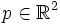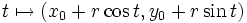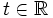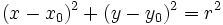# Circle in the plane

A generalization to higher dimensions is sphere in Euclidean space

## Definition

### General definition

Consider$\R^2$, the Euclidean plane. Let$p \in \R^2$ be a point and$r > 0$ be a positive real number. The circle with center$p$ and radius$r$ is the set of all points in$\R^2$ that have distance exactly$r$ from$p$.

Some easy facts:

• Two circles centered at the same point are termed concentric circles. Given two concentric circles, there is a dilation, or scaling, about the common center that takes one circle to the other
• Given two circles of the same radius but with different centers, there is a translation of$\R^2$ that sends one circle to the other. Namely, choose the translation that sends the center of the first circle, to the center of the second circle.
• The group of all orthogonal motions fixing the origin, sends each circle centered at the origin, to itself

### Variant definitions

In complex analysis, it is sometimes convenient to view a line as a circle. We think of the center of the line as being a point at infinity, and the radius as infinity. This makes the theory of inversion, the geometric intuition behind complex analysis, as well as coaxial systems of circles, easier to comprehend.

## Equational descriptions

### Parametric description

The circle can be viewed as a parametrized curve. In Cartesian coordinates, if the center of the circle is given by$(x_0,y_0)$, then the parametrization is:$t \mapsto (x_0 + r \cos t, y_0 + r \sin t)$

Here$t \in \R$, though we only need$t$ to vary over an interval of length$2\pi$ or more.

### Equational description

In Cartesian coordinates, the equational description of a circle with center$(x_0,y_0)$ and radius$r$ is given by:$(x -x_0)^2 + (y - y_0)^2 = r^2$

### Implicit description

Fill this in later

## The plane with respect to the circle

### Global study

The circle divides its complement in the Euclidean plane into two connected components. One component, called the interior of the circle, and also called an open disc, is the set of points that are at a distance from the center less than the radius. The other component, called the exterior of the circle, is the set of points whose distance from the center is greater than the radius.

The interior of a circle is contractible (in fact, we can contract it to the center by radial shrinking) whereas the exterior is not: it is homotopy-equivalent to the circle.

### Pointwise study

Some notions that come up when studying single points with respect to a circle include:

• Power of a point with respect to the circle
• Distance from the point to the center of the circle

Notions that relate multiple points:

### Points and lines

• Reciprocation is a map that sends points to lines and lines to points, sending a point to its polar and a line to its pole

## Families of circles

Important families of circles that come up: